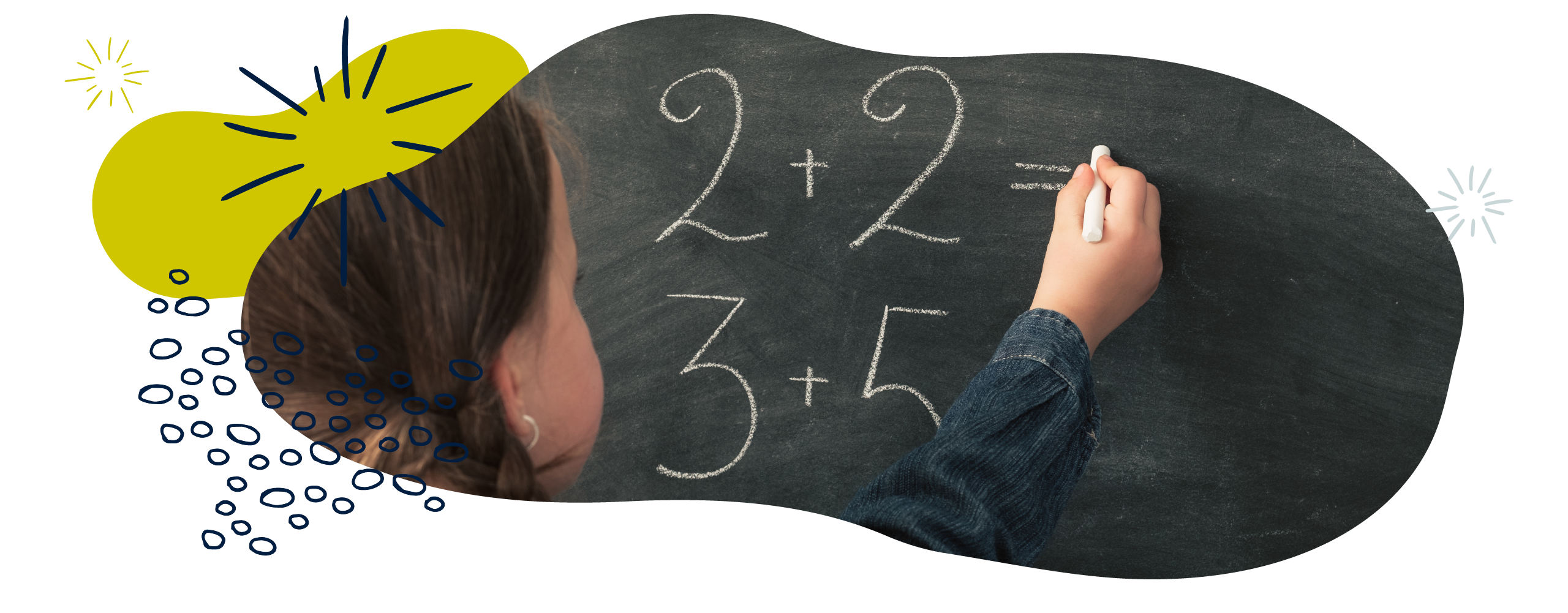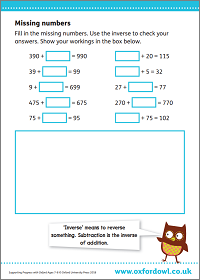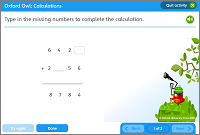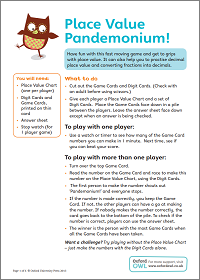We use cookies to enhance your experience on our website. By continuing to use our website, you are agreeing to our use of cookies. You can change your cookie settings at any time. Find out more# Addition & subtraction in Year 3 (age 7–8)

In Year 3, your child will use a range of strategies for calculating addition and subtraction problems. They will need to be able to explain how they have solved problems, especially when using mental maths.

The key words for this section are commutative, difference, and place value.

## What your child will learn

Take a look at the National Curriculum expectations for addition and subtraction in Year 3 (ages 7–8):

#### Add and subtract numbers mentally

Your child will need to mentally calculate sums involving:

• a three-digit number and 1s (for example, 234 +/– 7)
• a three-digit number and 10s (for example, 534 +/– 20)
• a three-digit number and 100s (for example, 635 +/– 300)

They will be taught lots of strategies to do this. It is good to use several strategies to check answers.

#### Add and subtract numbers with up to three digits

Your child will solve problems with a variety of methods, such as using objects (like counters, straws, and fruit), pictures (like number lines, grids, and arrays), and symbols (‘+’, ‘–’, and ‘=’).

Your child will also learn to use formal written methods such as column addition and column subtraction.

#### Estimate answers to calculations and use inverse operations to check answers

Your child will be expected to estimate answers to addition and subtraction problems before working out the answers accurately. They can do this by rounding numbers before a calculation. For example:

Estimate £5.73 + £13.12 + £2.14 = ?

Rounding to the nearest £1, the sum is £6 + £13 + £2 = £21.

So, when we work out the answer accurately, we can except the answer to be about £21. If it is not, we know that something has probably gone wrong with our calculation.

Your child will also use their understanding of the fact that addition and subtraction are inverse operations to check the accuracy of their answers. For example, if they have calculated 242 + 452 = 694, they could check the answer by working out that 694 – 242 = 452.

#### Solve complex problems using number facts and place value

Your child should now know several different ways to work out addition and subtraction problems. These include using place value, number facts, partitioning, and diagrams. They will need to be able to choose a method to solve a problem, and to check their answer using a different method.

Your child should be able to explain how they have solved a problem and why they have chosen a particular method. Using pictures and symbols can be a good way to explain your thought process.

## How to help at home

There are lots of quick and easy ways that you can help your child to understand addition and subtraction. Here are just a few everyday ideas to support your child’s learning:

### 1. Use the language of addition and subtraction

Using the correct mathematical vocabulary will get your child used to deconstructing word problems, and will make them familiar with the kinds of words they will hear in school.

Try to use mathematical language like add, altogether, more, plus, total, and sum when adding. When your child finds the answer to an addition calculation, encourage them to use the word total or sum. If they talk about the fact that you can add numbers in any order and get the same answer, encourage them to use the word commutative.

There is also a lot of mathematical vocabulary related to subtraction. When your child tells you the answer to a subtraction calculation, encourage them to refer to this as the difference. For example, ‘7 – 3 = 4’ could be read as ‘the difference between 7 and 3 is 4’. They should also try to use the language take away, subtract, minus, less, fewer, and so on.

Try writing out the addition and subtraction symbols (‘+’ and ‘–’) along with the related words on cards (for example, add, altogether, more, plus, total, sum, take away, subtract, minus, less, and fewer). Help your child to link the words to the correct symbol. If necessary, help them to read some of the words.

### 2. Try calculating mentally

It’s important for your child to develop their mental calculation skills. It is useful in everyday life, especially when technology (and paper) may not be available or appropriate, such as when you are shopping. Confidence in mental maths will help your child when making quick estimates and checking their calculations, too.

Encourage your child to use different methods when calculating mentally, such as counting on, counting back, and partitioning numbers. Your child should use their knowledge of place value, rounding, and inverse operations to make estimates and check their answers.

If your child is working on a problem and they announce the answer, ask them to explain how they solved the problem. They could draw pictures or diagrams to help them explain their method.

Ask your child to tackle the activities below using mental maths to keep their skills sharp!

### Activity: Missing numbersFill in the missing numbers.Fill in the missing numbers in these addition and subtraction calculations.

### 3. Play Place Value Pandemonium

Adding and subtracting becomes much easier if you have a good understanding of place value. Take a look at our Number & place value in Year 3 (age 7–8) page, or get to grips with place value with this fun, fast-paced game:

### Activity: Place value pandemoniumHave fun with this fast moving game and get to grips with place value.

### 4. Try lots of different methods

Encourage your child to use different methods for solving addition and subtraction problems. These could include:

• Partitioning numbers into parts to add or subtract. For example, to solve the problem 243 + 65, we could partition each number. Encourage your child to explain this to you, and perhaps model it to them first:
• ‘I partitioned 243 into 200, 40, and 3.
Then, I partitioned 65 into 60 and 5.
Then, I added the ones: 5 + 3 which equal 8.
Then, I added the tens: 40 + 60 = 100.
Then, I have the 200.
So, I added the 200 to the 100, and then added the 8, to get a total of 308.’

• Drawing pictures.
• Using number lines or hundred squares.
• Using written methods of column addition and subtraction.

Your child will understand subtraction as ‘taking away’ as well as ‘difference’. Methods could include:

• Placing groups of objects into two rows to compare and find the difference.
• Finding the difference between two numbers by counting back or counting up. For example, 27 – 18 could be interpreted as ‘what is the difference between 27 and 18?’ Your child may count back from 27 to 18 to find the difference of 9, or count up from 18 to 27 to find the difference of 9.

Encourage your child to partition numbers over 10 that you see around you – in shops, adverts, game scores, and so on. This kind of short, regular practice is perfect for getting them into the habit of calculating mentally.